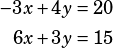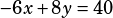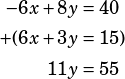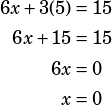Test 4 Section 3 Number 19

This one is a great opportunity to practice solving a system of linear equations by elimination. Here are the equations you’re given:Multiply both sides of the first equation by 2:Now add that to the original second equation and you’re left with only y terms!From that, you know that y = 5. The question asked you for x, though, so plug in 5 for y in one of the original equations to finish up: